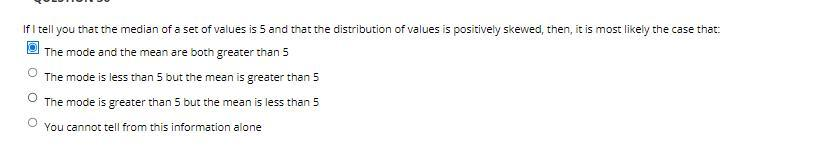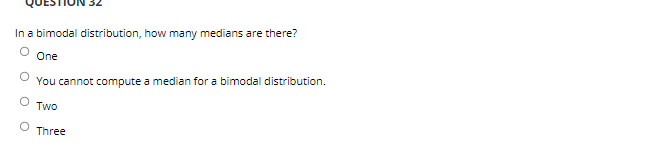Home / Expert Answers / Statistics and Probability / if-i-tell-you-that-the-median-of-a-set-of-values-is-5-and-that-the-distributi-pa720

# (Solved): If I tell you that the median of a set of values is 5 and that the distributi ...???????

If I tell you that the median of a set of values is 5 and that the distribution of values is positively skewed, then, it is most likely the case that: The mode and the mean are both greater than 5 The mode is less than 5 but the mean is greater than 5 The mode is greater than 5 but the mean is less than 5 You cannot tell from this information alone In a bimodal distribution, how many medians are there? One You cannot compute a median for a bimodal distribution. Two Three

We have an Answer from Expert